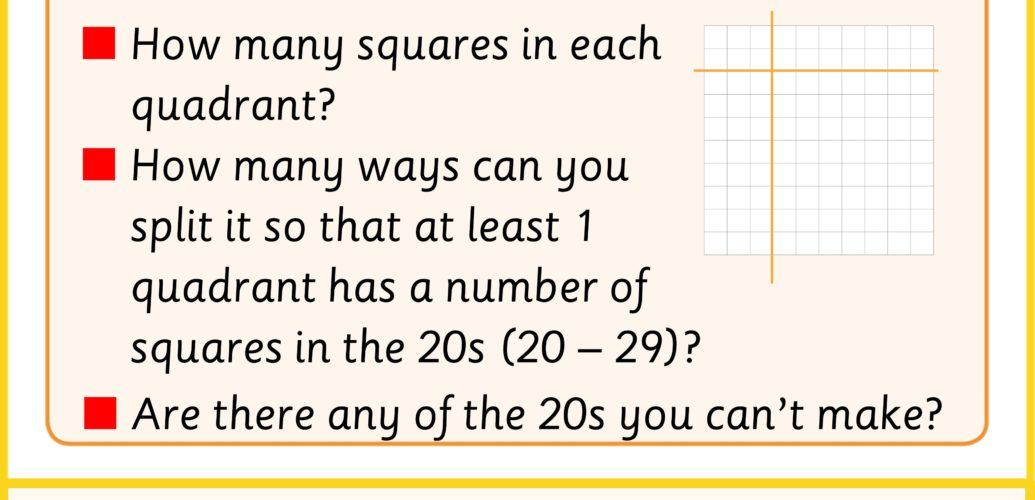### Challenge On Boarding – Orientation of Lines

The orientation of a line is the direction that the line goes in or could be considered as the angle that the line makes with the axes on a grid.

There are 3 common ways to describe the orientation of a line

• Vertical
• Horizontal
• Diagonal

We tend to use the words to mean roughly either aligned with the horizon (horizontal), perpendicular to the horizon (vertical) or somewhere in between (diagonal).

#### Lines, Rays and Line Segments

• A line segment is a line that has a starting point and an ending point
• A ray is a line that has a starting point and continues forever in a particular direction
• A line that goes on forever in both directions

### Challenge On Boarding – Quadrants

Quadrants are the result of splitting a rectangular shape into 4 pieces by making a vertical cut and a horizontal cut.

This is a conceptual tool that is used often to analyse real world situations.

The simplest version of this is a Carroll Diagram that can be used to sort items based on having or not having two separate attributes.  For example you could categorise fruits based on whether they are red (or not) and whether they are big (or not).

A Cartesian plane is an extension of this idea.  This extends the binary nature of the Carroll diagram to include a scale.  This is a really important idea in mathematics.

### Challenge On Boarding – Arrays and the Area Model

The area model is one of the most powerful ways to start getting an understanding of multiplication.  It can help us understand multiplication of natural numbers (1, 2, 3 etc.) as well as fractional numbers.

This is based on the idea of an array.  The arrays on the left can be used to represent 3 groups of 5 or 5 groups of 3.  This is how multiplication can be understood.

An rectangular area can be thought of as an array of squares, but can be used to extend the idea to fractional numbers too.

This means we can use multiplication to count items in larger arrays or areas.

### The Challenges

1. Split the grid and count the squares
2. Find quadrants with amounts of squares in the 20s
3. Find ways to split the grids to give you particular numbers

Download or share all of these challenges with this pdf and let us know how you got on with it by tweeting using #momentofmaths and start to see Every moment is a #momentofmaths.